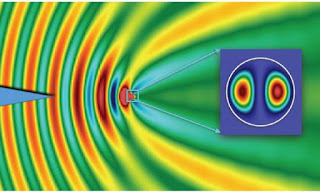## Thursday 20 February 2020

### Exercise 1.12 Energy momentum tensors of two field theoriesEM waves by Lomonosov Moscow State University
Question
Consider the two field theories we explicitly discussed, Maxwell's electromagnetism (let $J^\mu=0$) and the scalar field theory defined by (1.148)

(a) Express the components of the energy-momentum tensors of each theory in three-vector notation, using divergence, gradient, curl, electric and magnetic fields, and an overdot to denote time derivatives.

(b) Using the equations of motion, verify (in any notation you like) that the energy-momentum tensors are conserved.

The energy momentum tensors in question were
\begin{align}
T_{scalar}^{\mu\nu}=\eta^{\mu\lambda}\eta^{\nu\sigma}\partial_\lambda\phi\partial_\sigma\phi-\eta^{\mu\nu}\left(\frac{1}{2}\eta^{\lambda\sigma}\partial_\lambda\phi\partial_\sigma\phi+V\left(\phi\right)\right)&\phantom {10000}(1)\nonumber
\end{align}and
\begin{align}
T_{EM}^{\mu\nu}=F^{\mu\lambda}F_{\ \ \ \lambda}^\nu-\frac{1}{4}\eta^{\mu\nu}F^{\lambda\sigma}F_{\lambda\sigma}&\phantom {10000}(2)\nonumber
We had to answer a and b for each tensor so that makes four questions really.

a scalar) The first one was quite easy but we had to use the outer product $\bigotimes$ and the Kronecker delta $\delta^{ij}$ too. (Assuming $\nabla\phi\bigotimes\nabla\phi$ means what I think it means.)

a TM) The second was quite difficult and I found a new tool Microsoft Mathematics to multiply horrid matrices which come from the likes of $F^{\mu\lambda}F_{\ \ \ \lambda}^\nu$ in (2).

In both the b cases we need to show that the tensors are conserved we just have to show that
\begin{align}
\partial_\mu T^{\mu\nu}=0&\phantom {10000}(3)\nonumber
\end{align}which is four equations one for each $\nu$, the four coordinates.

b scalar) I was able to do that entirely by tensor and index manipulation which was cool. The time and space coordinates worked the same.

b EM) This was a horrible mixture of tensor and index manipulation and then decomposition into electric and magnetic fields. I had to do the $t,x$ coordinates separately and assumed that  $y,z$ are like $x$. It was nasty.

Read the full answer here: Ex 1.12 Energy momentum tensors of two field theories.pdf

#### 1 comment:

1.There are a few sign errors in your solution. The most important one, conceptually, is in (8), where grad(φ)^2 should have a positive sign.